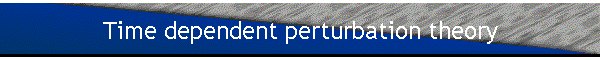Assume that at t=-¥ a system is in an eigenstate |fi> of the Hamiltonian H0.  At t=t1 the system is perturbed and the Hamiltonian becomes H=H0+W(t).  When investigating the interaction picture we found that the probability of finding the system in the eigenstate |ff> of the Hamiltonian H0 at t=t2 is given by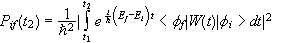to first order in the perturbation W.

We often write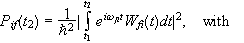This is the result of first order time dependent perturbation theory.

• Assume that W(t)=Wsinwt, i.e. that we have a sinusoidal perturbation starting at t=0. Then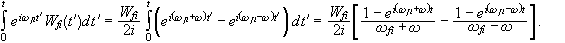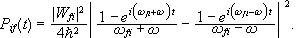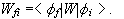• Similarly, if W(t)=Wcoswt, then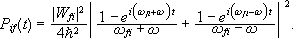• If w=0 we have a constant perturbation and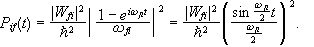For the harmonic perturbation W(t)=Wsinwt, we find that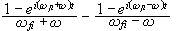has an appreciable amplitude only if the denominator of one of the two terms is approximately zero, i.e. if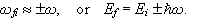The first order effect of a perturbation that varies sinusoidally with time is to receive from or transfer to the system a quantum of energyIf the system is initially in the ground state, then Ef>Ei, and only the second term needs to be considered. Then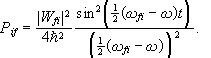Let b=wfi-w, and plot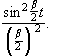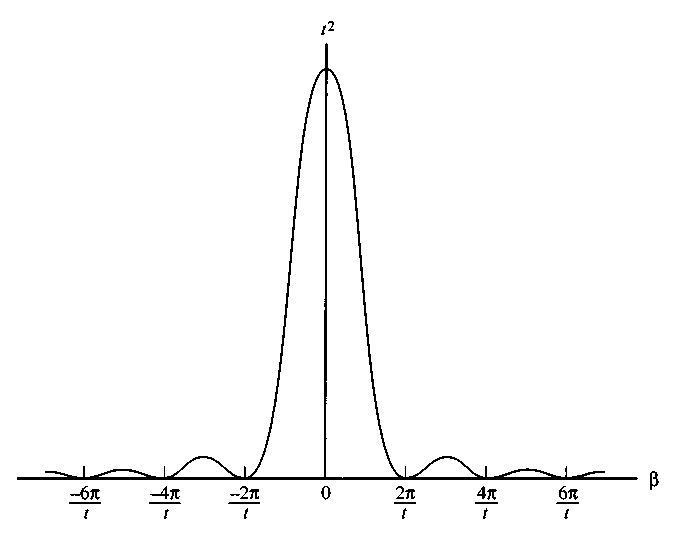If b=0, i.e. wfi=w, then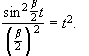Therefore, if wfi=w, then the probability of finding the system in the state |ff>,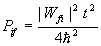increases linearly with time.

In the above figure the height of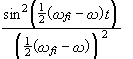increases proportional to t2, and the width of the peak is proportional to 1/t.  The area under the curve is proportional to t.

For a first order approximation to be valid, we need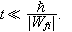On the other hand, to justify neglecting the first term in the above formula, we need 2wfi>>Dw. 2wfi is the difference in the positions of the peaks due to the first term and the second term in the above formula, Dw is the width of the peaks, Dw»4p/t.  We therefore need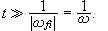Combining these two conditions we obtain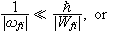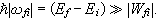Assume there is a group of states n, nearly equal in energy E, and that Wni=<fn|W|fi> is nearly independent of n for these states.  Take for example continuum states.  We may label continuum states by |a>, where a is continuous. <a|a>=d(a-a').  The probability of making a transition to one of these states in a small range Da is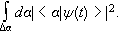If |a>=|b,E> then da=r(b,E)dE, where r(b,E) is the density of states.  We assume b to be some discrete index.  We then have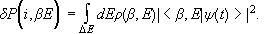If W is a constant perturbation, then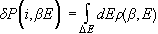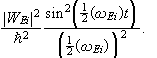The function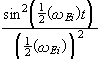peaks at wEi=0 and has an appreciable amplitude only in a small interval DwEi or DE about wEi=0.  We assume that r(b,E) and |WEi|2 are nearly constant in that small interval and therefore may be taken out of the integral.  Then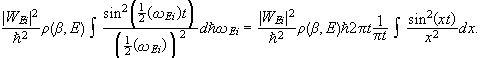Therefore,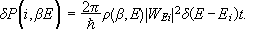It is understood that the expression is integrated with respect to dE.

The transition probability per unit time is the given by Fermi’s golden rule,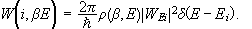Similarly, for a sinusoidal perturbation W(t)=Wsinwt or W(t)=Wcoswt we obtain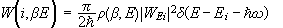and for W(t)=Wexp(±iwt) we obtain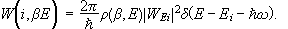#### An atom interacting with a monochromatic plane electromagnetic wave

Let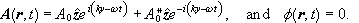We have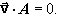(Coulomb or radiation gauge)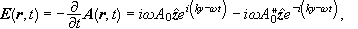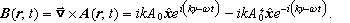We have a plane electromagnetic wave propagating in the y-direction.

Let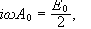and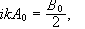with E0 and B0 real,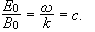( SI units ) ThenS is the Poynting vector.

The Hamiltonian of an atomic electron interacting with this plane wave isIn this expression we consider only one independent electron, and we neglect the spin orbit interaction.  We have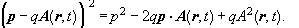[pz,Az]=0 since Az depends only on y.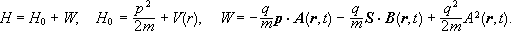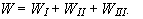To find induced transition probabilities, we have to evaluate the matrix elements of W(t) between unperturbed bound states.  An order of magnitude estimate reveals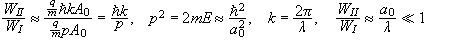in the optical domain.  We assume that the intensity of the wave is low enough so that WIII, the term containing A02, can be neglected compared to terms containing A0.

#### Evaluating the matrix elements of WI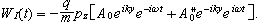since y is on the order of atomic dimensions.

Let WDE(t) be the zeroth order term in the expansion.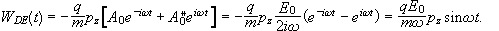WDE is called the electric dipole Hamiltonian.  The electric dipole approximation assumes W(t)=WDE(t).

Note: This form of WDE is equivalent to the form we would get starting with the energy of an electric dipole in an electric field.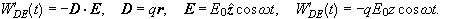This equivalence can be shown via a gauge transformation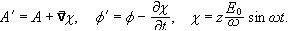Let us now examine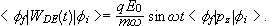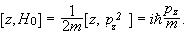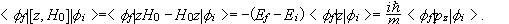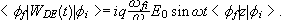The matrix elements of WDE are proportional to the matrix elements of z, because E is in the z direction.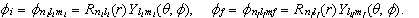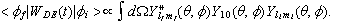The integrant is a product of three spherical harmonics and the integral can be given in terms of Clebsch Gordan coefficients.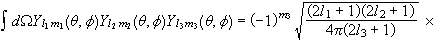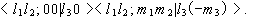These are coefficients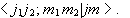The integral is zero unless

• i)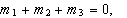• ii)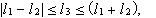• iii)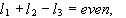since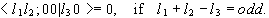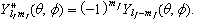We therefore have that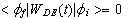unless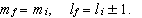If we choose another direction for the polarization of E, i.e.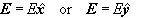then we find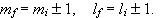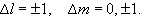These selection rules result as a consequence of the properties of the spherical harmonics.

An electromagnetic field is most likely to induce a transition between an initial and a final state if these selection rules are satisfied.  If these selection rules are not satisfied a transition is less likely and is said to be forbidden.

When deriving the dipole transition selection rules Dl=±1, Dm=0, ±1, we assumed that the Hamiltonian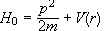was perturbed by WDE(t).  We neglected the spin orbit interaction.  If H0 contains a spin orbit coupling term f(r)L·S, then the eigenstates of H0 are

{|l,s;j,mj>} and not {|l,s;m,ms>}.  The dipole selection rules then become Dj=0, ±1, (except ji=jf=0), Dl=±1, Dmj=0, ±1.

(p or r are vector operators.  The selection rules follow from the Wigner-Eckart theorem.)

#### Higher order terms: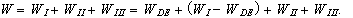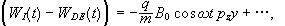if we take the first order term in the expansion of exp(±iky).  We may write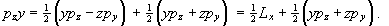If in the expression for WII(t) we only keep the zeroth order term in the expansion of exp(±iky),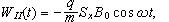then we are looking at terms of similar magnitude.  We write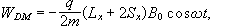called the magnetic dipole Hamiltonian, and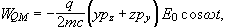called the electric quadrupole Hamiltonian.

Transition induced by WDM obey the magnetic dipole transition selection rules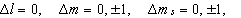or, if H0 includes a spin orbit coupling term,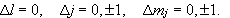Transition induced by WQM obey the electric quadrupole transition selection rules